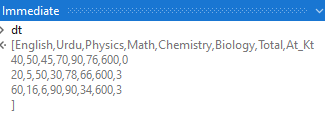# How to find out the Column values are less than 40

Hi,
How to find out the below marked subject is less than 40.
if less than 40 then count could be 1, 2, 3 etc

Kindly assist.

@VJK

Welcome to forums

You can use Write cell activity and write CountIF expression

Check below for CountIF

Thanks

Hi @VJK ,

I do think there is Still not enough information as to How you would require the Output to appear.

Could you also provide the Expected Output for the Input Excel Shown ?

Hi @VJK ,

Assuming that you want to assign the count of failed subjects to At_Kt Column, you may use an Invoke Code to achieve it →``````dt.AsEnumerable().ToList().ForEach(sub(row) row("At_Kt")={"English", "Urdu", "Physics", "Math", "Chemistry", "Biology"}.Select(Function(s) If(CInt(row(s).ToString)<40,1,0)).Sum)
``````

Benefit of using an Invoke Code is that you don’t have to worry about specifying all columns - you can simply focus on those columns that you want to work with.

GetBelowFortyCount.xaml (8.4 KB)

Kind Regards,
Ashwin A.K

This is look like hardcoded.

Hi @VJK ,

Have you tested the sequence shared above?
Replace the Build DataTable Activity with a Read Range Activity and please let me know if it works as expected.

Kind Regards,
Ashwin A.K

@Ashwin,
Not working as expected.
I am sharing the sheet,
Kindly help to print number of count in Al_KL columns if subject mark is less than 40
and rank will be based on mark.

Hi @VJK ,

We’d appreciate it if you could provide the sample dataset so that we can create a proper end to end resolution for you, could you please do the needful?

Kind Regards,
Ashwin A.K

@Ashwin,
I am unable to upload file here due to new user.

Given below the format for data set and expected result in Al_KT and Rank columns

Hi @VJK ,

Could you give this a try?

``````Dim arr_subjects = {"English", "Physics", "Chemistry", "CS"}
Dim arr_marks = dt.AsEnumerable().Select(Function(s) arr_subjects.Sum(Function(su) CInt(s(su).ToString))).OrderByDescending(Function(o) o).ToArray()
dt.AsEnumerable().ToList().ForEach(Sub(row)
row("at_kt")=arr_subjects.Select(Function(s) If(CInt(row(s).ToString)<40,1,0)).Sum
row("Rank") = Array.IndexOf(arr_marks,arr_subjects.Sum(Function(su) CInt(row(su).ToString)))+1
End Sub)
``````

GetBelowFortyCount_v1.xaml (8.4 KB)
Kind Regards,
Ashwin A.K